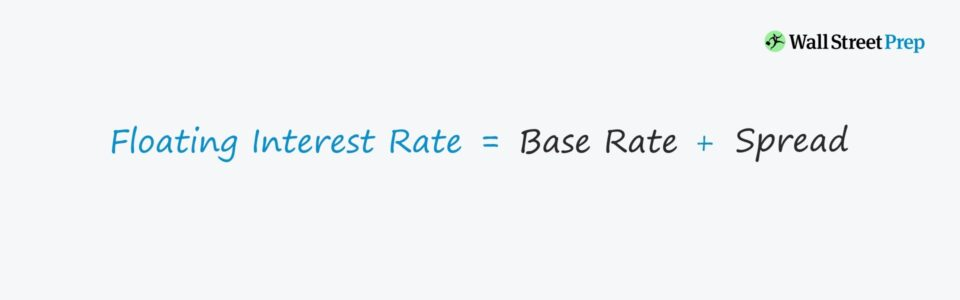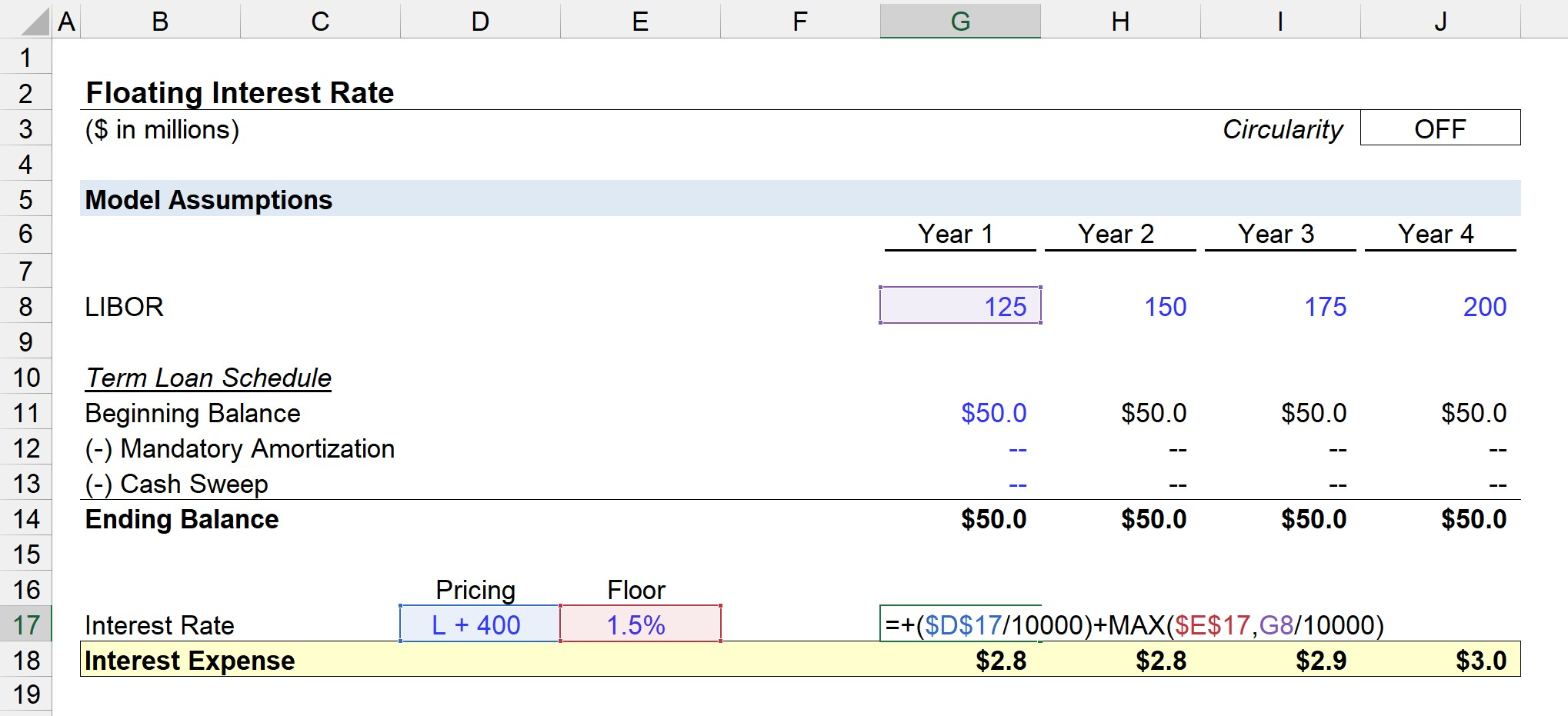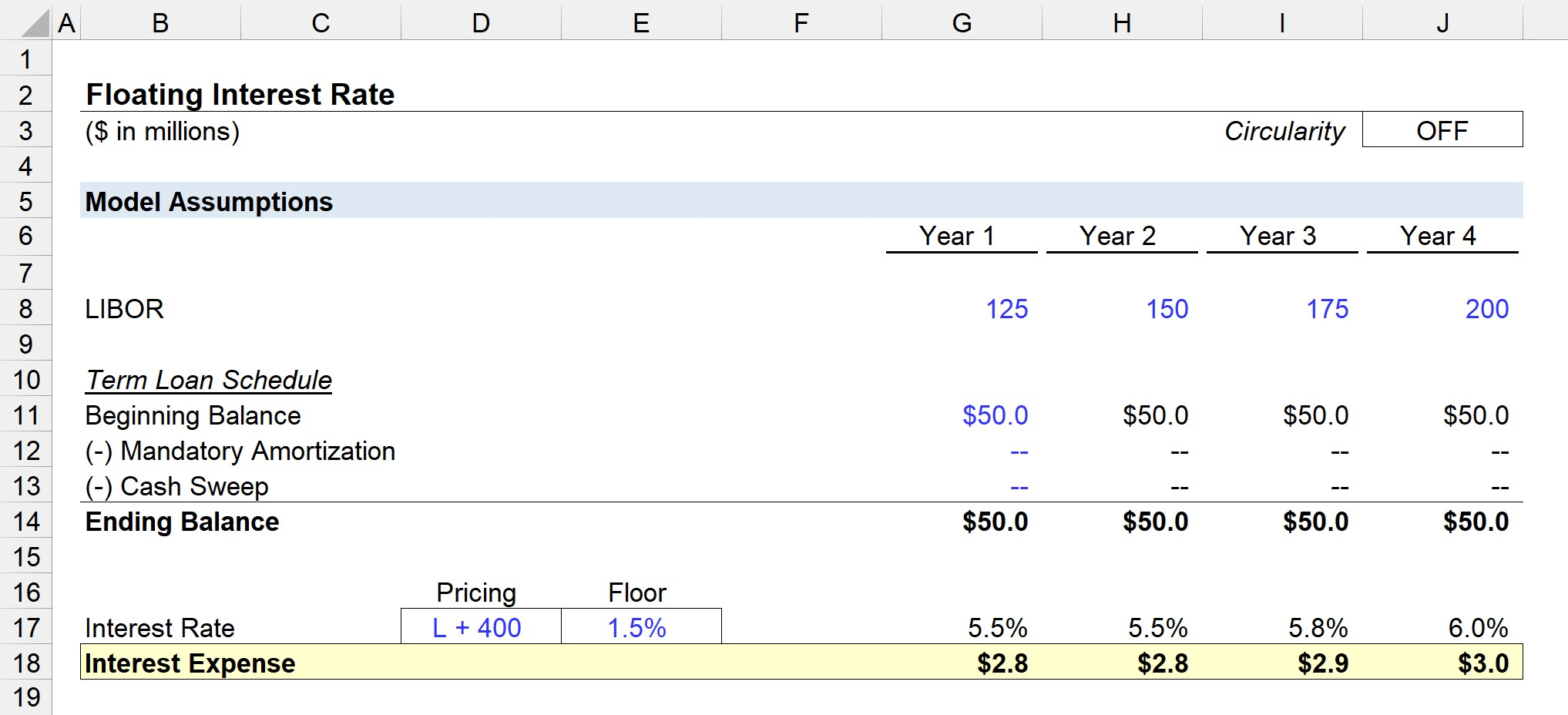# Floating Interest Rate

Guide to Understand Floating Interest Rates## How to Calculate Floating Interest Rate (Step-by-Step)

A floating interest rate, often called a “variable rate”, is when a debt instrument is priced at a rate contingent on an underlying benchmark.

The interest rate attached to debt is defined as the amount charged to the borrower by the lender periodically throughout the borrowing term and is expressed as a percentage of the outstanding loan amount.

Unlike fixed interest rates, which remain constant throughout the entire duration of the borrowing, floating interest rates fluctuate based on the prevailing economic conditions.

The interest rate pricing of debt with floating interest rates is typically expressed in two parts:

• Base Rate (e.g. LIBOR)

## Floating Interest Rate Formula

The formula for calculating the interest expense on securities priced on a variable basis is as follows.

Floating Interest Rate (%) = Base Rate + Spread

Generally speaking, floating interest rates are associated with senior debt, whereas fixed interest rates are far more common with bonds and riskier forms of debt securities.

## LIBOR Debt Pricing Example

Historically, the standard benchmark for borrowings has been LIBOR, which stands for the “London Inter-Bank Offered Rate”.

LIBOR is the rate at which financial institutions lend to one another for overnight, short-term loans.

Interest Rate = LIBOR + Spread

Let’s say that LIBOR – the basis of a debt’s pricing – is currently at 150 basis points, and a senior loan’s interest rate is “LIBOR + 400”.

In this case, the interest rate on the loan (i.e. the cost of the borrowing), is equal to 5.5%.

• Interest Rate = (150 / 10,000) + (400 / 10,000)
• Interest Rate = 1.5% + 4.0% = 5.5%

Side note: LIBOR is gradually being phased out and is expected to be replaced by Secured Overnight Financing Rate (SOFR) by the end of 2021. The process of the LIBOR phase-out is anticipated to be fully complete by 2023.

## Floating Interest Rate vs. Fixed Interest Rate

A fixed interest rate – as implied by the name – is a rate that remains constant during the entire lending period.

That said, fixed interest rates are independent of any market-based benchmark.

By contrast, a floating interest rate moves up and down based on the movements of the underlying index (e.g. LIBOR, SOFR).

The impact of changes in the market rate are as follows:

• Falling Market Rate → Beneficial for the Borrower (i.e. Lower Interest Rate)
• Rising Market Rate → Not Beneficial for the Borrower (i.e. Higher Interest Rate)

From the perspective of both parties – the lender and the borrower – floating interest rates come with more risk due to potentially unpredictable changes in the benchmark.

The advantages of a floating interest rate come at the expense of one party, either the borrower or lender. For example, when rates are low, the borrower benefits, but when rates are high, the lender benefits (and vice versa).

However, as a protective measure for the lender, an interest rate “floor” is typically included to ensure a certain minimum yield is received – which means if the underlying benchmark (e.g. LIBOR) falls below a specified value, the greater between the two is selected:

1. Benchmark Rate
2. Floor Rate

### The Wharton Online and Wall Street Prep Private Equity Certificate Program

Level up your career with the world's most recognized private equity investing program. Enrollment is open for the Sep. 5 - Oct. 27 cohort.

## Floating Interest Rate Calculator – Excel Template

We’ll now move to a modeling exercise, which you can access by filling out the form below.Submitting ...

## Floating Interest Rate Calculation Example

For our illustrative scenario, we’ll assume there is a term loan with an outstanding balance of \$50 million.

For purposes of simplicity, there is neither any mandatory amortization nor a cash sweep.

As a result, the \$50 million term loan balance remains constant across all four periods.

To calculate the interest rate, the spread is added to the LIBOR in the corresponding year, as shown below in the screenshot.From above, we can also see the “MAX” function in Excel is used to ensure that the LIBOR value used in the calculation does NOT dip below the interest rate floor of 1.5%.

Hence, the interest rate is 5.5% for the first two years (i.e. spread + the minimum floor), but when LIBOR exceeds 150 basis points, the rate increases to 5.8% and 6.0% in the subsequent years, respectively.

Note that LIBOR and the pricing are denoted in basis points, so we must divide each figure by 10,000 to convert to a percentage.

Upon multiplying the interest rate by the average of the beginning and ending balance of the term loan, we arrive at the interest expense charged in each period – which increases from \$2.8 million to \$3.0 million during the projection period as a result of the increase in LIBOR.Step-by-Step Online Course

### Everything You Need To Master Financial Modeling

Enroll in The Premium Package: Learn Financial Statement Modeling, DCF, M&A, LBO and Comps. The same training program used at top investment banks.Inline FeedbacksLearn Financial Modeling Online

Everything you need to master financial and valuation modeling: 3-Statement Modeling, DCF, Comps, M&A and LBO.

X

The Wall Street Prep Quicklesson Series

7 Free Financial Modeling Lessons

Get instant access to video lessons taught by experienced investment bankers. Learn financial statement modeling, DCF, M&A, LBO, Comps and Excel shortcuts.